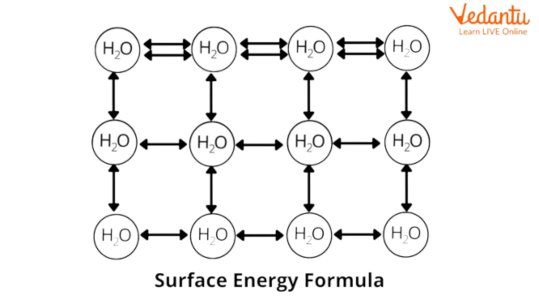Courses
Courses for Kids
Free study material
Free LIVE classes
More

# Surface Energy - JEE Important TopicLIVE
Join Vedantu’s FREE Mastercalss

## Introduction to Surface Energy

Surface free energy or interfacial free energy quantifies the breaking of intermolecular bonds that occurs when a surface is created. Solid-state physics requires that the surface itself be energetically more favorable than the bulk of the material. Surface energy can therefore be defined as the excess energy at the surface of a material compared to the bulk. The "excess energy" exists because the bonding at the two surfaces is currently imperfect and unrealized.## What is Surface Energy?

Surface energy is the work performed at the outer area of a material in which the atoms do not have a bond with another atom of their immediate neighborhood. It is vital that the atoms in any fabric are bonded with different atoms. This is because the majority of the unchanged physical element of the material is totally surrounded through bonded atoms. However, while the material is nearing the end and reaching the surface, the bonds of the atom split open, and there are no bonds at the outer surface of the material. This is referred to as surface energy.## Surface Energy Formula:

${\text{Surface}}~{\text{Energy}} = \dfrac {\text{Energy}}{\text{Area}}$

${\text{Surface}}~{\text{Energy}}=E = S \times \Delta {A}$

where in,

S = surface tension and

ΔA = upward thrust in surface place.

The power retained by way of means of the liquid surface is referred to as surface power. Variation in surface energy is the material from Surface Tension and variance withinside the surface place below the consistent temperature. The peak to which water will increase in a capillary tube of radius r is calculated.

## Dimension of Surface Energy:

SI Unit and size of Surface energy is given withinside the desk below.

• S.I. Unit N/m

• Dimension [MT-2]

## Relation Between Surface Tension and Surface Energy

Let us derive a relation among the Surface Tension and Surface energy.

Due to this Surface Tension is given with the aid of using,

$F = 2T l$ ………..(1),

Where

T - Surface Tension of the cleaning soap movie

l - Length

For slowly transferring the slider outwards, the outside pressure appearing on it could be:

${F_{ext}}= 2Tl$……….(2)

Where,

T - Surface Tension of the cleaning soap movie

l - Length of the slider

Now, allow us to displace the slider with the aid of using Δx distance outwards, then there could be a few paintings carried out on it.

Whatever paintings are carried out to transport the slider could be saved withinside the shape of its ability electricity. Therefore, the paintings carried out in displacing the slider is given with the aid of using:

$dW = {F_{ext}} \nabla x$

$\nabla U = dW = 2Tl .\nabla x$

Where,

$\nabla U$ - Increase withinside the Surface energy

On rearranging the above expression,

$\nabla U$ = T \nabla S $Where,$\nabla S $- Increase in surface energy Therefore, the relation among Surface Tension and Surface energy is given with the aid of using the subsequent expression: T =$ \nabla U. \nabla S \$ ……….(5)

Equation (5) offers the relation among Surface Tension and Surface energy.

## Conclusion

In this article, we have studied about surface energy in detail. Surface energy plays a crucial part in solids and liquid. We have covered the surface energy definition along with the mathematical formula and dimensional analysis. The relation between the surface tension and the surface energy was established using a mathematical approach.

Last updated date: 26th Sep 2023
Total views: 2.1k
Views today: 0.02k

## FAQs on Surface Energy - JEE Important Topic

1. What is the difference between surface tension and surface energy?

Surface energy and Surface Tension are measurements of intermolecular forces that incorporate material. Because of those intermolecular forces, a surface of a liquid is continually being pulled or dragged inside. If one is stretching the surface, paintings have to be achieved so as to conquer intermolecular forces. The tension at the surface of a liquid and the quantity of labor required to stretch that surface may be measured, and those measurements correspond to the Surface energy and the Surface Tension. The predominant distinction among Surface energy and Surface Tension is that Surface energy is used to degree the quantity of energy that is required to be achieved in keeping with unit location to stretch it despite the fact that Surface Tension is used to degree pressure in keeping with unit period of the surface.

2. What are the uses of surface tension in daily life?

Surface tension plays a major role in a variety of common phenomena, including the adhesion of raindrops to windows, the formation of bubbles in sinks when you add detergent, and the propulsion of insects on pond surfaces. At the same time, surface tension is also an important element in industrial operations. Surface tension phenomenon is used by R&D departments in all industrial plants to raise product quality. The quality of the product, including detergent formulas, is improved by a variety of activities.